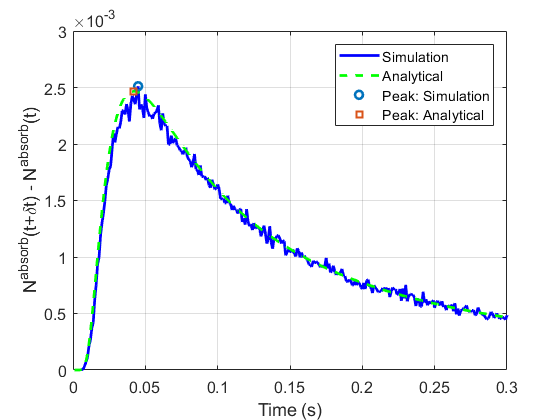# #StackBounty: #distributions #brownian #diffusion What is the distribution of the peak time of the first hitting time process

### Bounty: 50

I need to find the distribution of the random variable $$T_{peak}$$ where $$T_{peak}$$ represents the peak time of the first hitting time process.

Detailed Explanation of the System:
There are emitted molecules from a specific point in 3D environment. Molecules diffuse in the environment according to the followings:

$$r[t] = r[t-1] + (Delta r_1, Delta r_2, Delta r_3)$$
$$Delta r_i sim mathcal{N}(0,, 2DDelta t)$$
where $$r[t]$$, $$r_i$$, $$D$$, and $$Delta t$$ are the location vector at time $$t$$, $$i$$-th component of the location vector, diffusion coefficient, and the time step, respectively.

If there is an absorbing spherical trap at a distance $$d$$, the mean number of arriving/hitting molecules until time $$t$$ is:

$$E[N^{absorb}(t)] = frac{r_{trap}}{d+r_{trap}} , text{erfc} left( frac{d}{sqrt{4Dt}} right) = frac{r_{trap}}{d+r_{trap}} , 2Phi left( frac{-d}{sqrt{2Dt}} right)$$
where $$r_{trap}$$ is the radius of the absorbing spherical trap.

When you plot $$N^{absorb}(t)$$ in small intervals, you get something like the following figure (Scaled Inverse Gaussian distribution)And the expected value of the peak time of hitting time histogram is
$$E[T_{peak}] = frac{d^2}{6D}$$

When simulating this diffusion process and the focusing on the absorbing times, $$T_{peak}$$ differs from simulation instance to instance, $$T_{peak}$$ is a random variable and I need to find the distribution of $$T_{peak}$$.

P.S. These absorbed molecules are considered as the received signal in molecular communications and the peak time distribution of the received signal is important for many applications.

Get this bounty!!!

This site uses Akismet to reduce spam. Learn how your comment data is processed.